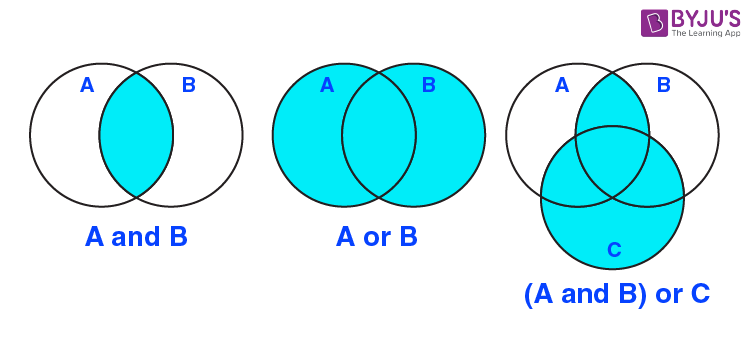# Compound Statements In Mathematics

Mathematical reasoning is a deductive process and its basic entity is a statement. The statements in reasoning can be compound i.e. they can be composed of two or more than two statements together. To frame compound statements certain special words or phrases like And, Or etc. are used in mathematical reasoning questions. These words are known as connectives.Let us discuss the basic connectives to study statements properly.

## Compound Statement Using Connective “And”

Rules regarding the use of connective “And”

(i) If all the component statements connected by ‘and, are true then the given statement is also true.

(ii) If any one of the component statements connected by the connective ‘and’ is false; then the entire compound statement is false.

Consider the following statement:

P: A square has four sides and all its sides are equal.

This statement joins two different mathematically acceptable statements and takes the connective and. If we split this statement into component statements we have;

a: A square has four sides

b: All sides of a square are equal

As both the component statements are true mathematically, therefore, the statement P is also true.

Example:

Let us take another example to understand it in a better way:

P: The squares of 3, 4, 5 and 6 are 9, 16, 25 and 36 respectively.

This statement P can be split as:

a: The square of 3 is 9.

b: The square of 4 is 16.

c: The square of 5 is 25.

d: The square of 6 is 36.

These component statements when put together give us the statement P.

But it can be seen that all components are correct hence as the given statement takes the connective “and”.

So, the given compound statement is true.

## Compound Statement Using Connective “Or”

Rules regarding the use of connective “Or”

(i) If any of the component statements connected by Or is true then the given compound statement is also true.

(ii) If all of the component statements are connected by the connective and are false then the entire statement is false.

Let us have a look at the following statements:

P: The sum of two integers can be positive or negative.

The component can be given as:

a: The sum of two integers can be positive

b: The sum of two integers can be negative

As the statement uses Or as a connective then if either of the statements is true then P is true. Here both a and b are true therefore P is true.

The Or connective can be inclusive or exclusive.

Example:

P: You can go in the east direction or west direction.

This means you can only choose one direction either east or west but not both. This is exclusive Or

S: The candidates who have scored 75% or 8 pointers are eligible for the job

This statement is inclusive Or.

We have seen what connectives in mathematical reasoning are. Please log on to www.byjus.com and enjoy the experience of learning.